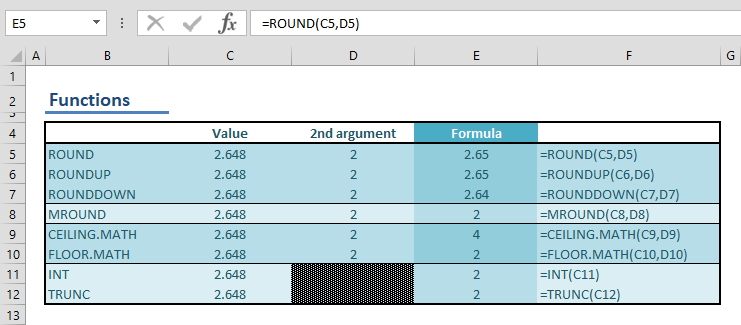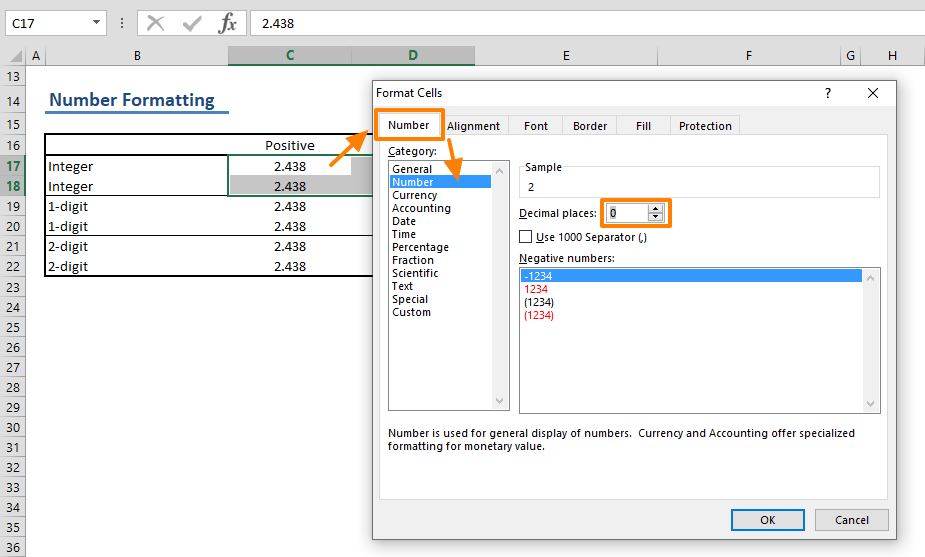Rounding numbers is a crucial element of creating legible and easy-to-use spreadsheet tools. Excel offers a few methods to do this, including a formula approach and the number formatting feature. In this guide, we're going to show you how to round numbers in Excel with and without the use of formulas.

## Rounding numbers using formulas

Excel has a handful of formulas when it comes to rounding formulas. The formulas below can be used to round a number to any number of digits:

The formula below can round a number to the specified multiple:

These two formulas can round a given number, to the nearest multiple of significance:

This one can round a number down to the nearest integer:

Finally, this one doesn't actually round the number, but decouples the integer part:## The Number Formatting feature

The dedicated functions allow for a dynamic approach when it comes to rounding numbers. However, the functions override the actual numeric value. On the other hand, if you want to display a rounded value while keeping its original value, you will need to use number formatting instead. The downside of the number formatting is the lack of variety. You need to stick with the "decimal" rounding, and don't have the option to define the rounding direction. The number is displayed as is, rounded to nearest specified digit. For example, while 2.43 becomes 2.4, 2.46 becomes 2.5.

Follow the steps below to round numbers using the number formatting feature:

1. Select the cell(s) which you want to round
2. Press Ctrl + 1 to open the Format Cells window
3. Make sure the Number tab is active
4. Under the Category section, select Number: The number category gives you an option for the number of the decimals you want to display.
5. Set a number for decimals. For example, set 0 to round your number to the nearest integer.
6. Click OK to apply.